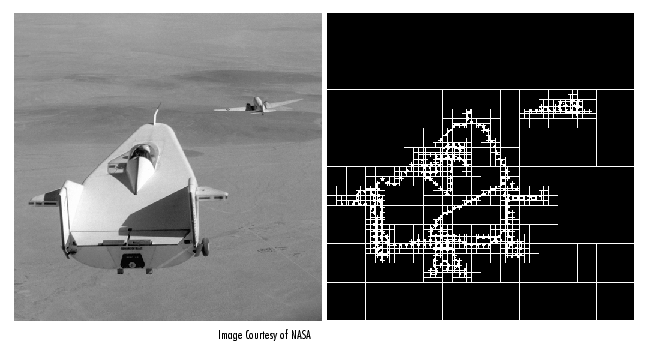Image Processing Toolbox User's Guideqtdecomp

Syntax

• ```S = qtdecomp(I)
S = qtdecomp(I,threshold)
S = qtdecomp(I,threshold,mindim)
S = qtdecomp(I,threshold,[mindim maxdim])
S = qtdecomp(I,fun)
```

Description

`qtdecomp` divides a square image into four equal-sized square blocks, and then tests each block to see if it meets some criterion of homogeneity. If a block meets the criterion, it is not divided any further. If it does not meet the criterion, it is subdivided again into four blocks, and the test criterion is applied to those blocks. This process is repeated iteratively until each block meets the criterion. The result can have blocks of several different sizes.

`S = qtdecomp(I)` performs a quadtree decomposition on the intensity image `I` and returns the quadtree structure in the sparse matrix `S`. If `S(k,m)` is nonzero, then `(k,m)` is the upper left corner of a block in the decomposition, and the size of the block is given by `S(k,m)`. By default, `qtdecomp` splits a block unless all elements in the block are equal.

`S = qtdecomp(I,threshold)` splits a block if the maximum value of the block elements minus the minimum value of the block elements is greater than `threshold`. `threshold` is specified as a value between 0 and 1, even if `I` is of class `uint8` or `uint16`. If `I` is `uint8`, the threshold value you supply is multiplied by 255 to determine the actual threshold to use; if `I` is `uint16`, the threshold value you supply is multiplied by 65535.

`S = qtdecomp(I,threshold,mindim)` will not produce blocks smaller than `mindim`, even if the resulting blocks do not meet the threshold condition.

`S = qtdecomp(I,threshold,[mindim maxdim])` will not produce blocks smaller than `mindim` or larger than `maxdim`. Blocks larger than `maxdim` are split even if they meet the threshold condition. `maxdim/mindim` must be a power of 2.

`S = qtdecomp(I,fun)` uses the function `fun` to determine whether to split a block. `qtdecomp` calls `fun` with all the current blocks of size `m`-by-`m` stacked into an `m`-by-`m`-by-`k` array, where `k` is the number of `m`-by-`m` blocks. `fun` returns a logical `k`-element vector, whose values are 1 if the corresponding block should be split, and 0 otherwise. (For example, if `k(3)` is 0, the third `m`-by-`m` block should not be split.) `fun` must be a `function_handle`.

Class Support

For the syntaxes that do not include a function, the input image can be of class `logical`, `uint8`, `uint16`, `int16`, `single`, or `double`. For the syntaxes that include a function, the input image can be of any class supported by the function. The output matrix is always of class `sparse`.

Remarks

`qtdecomp` is appropriate primarily for square images whose dimensions are a power of 2, such as 128-by-128 or 512-by-512. These images can be divided until the blocks are as small as 1-by-1. If you use `qtdecomp` with an image whose dimensions are not a power of 2, at some point the blocks cannot be divided further. For example, if an image is 96-by-96, it can be divided into blocks of size 48-by-48, then 24-by-24, 12-by-12, 6-by-6, and finally 3-by-3. No further division beyond 3-by-3 is possible. To process this image, you must set `mindim` to 3 (or to 3 times a power of 2); if you are using the syntax that includes a function, the function must return 0 at the point when the block cannot be divided further.

Example

• ```I = uint8([1 1 1 1 2 3 6 6;...
1 1 2 1 4 5 6 8;...
1 1 1 1 7 7 7 7;...
1 1 1 1 6 6 5 5;...
20 22 20 22 1 2 3 4;...
20 22 22 20 5 4 7 8;...
20 22 20 20 9 12 40 12;...
20 22 20 20 13 14 15 16]);

S = qtdecomp(I,.05);
disp(full(S));
```

View the block representation of quadtree decomposition.

• ```I = imread('liftingbody.png');
S = qtdecomp(I,.27);
blocks = repmat(uint8(0),size(S));

for dim = [512 256 128 64 32 16 8 4 2 1];
numblocks = length(find(S==dim));
if (numblocks > 0)
values = repmat(uint8(1),[dim dim numblocks]);
values(2:dim,2:dim,:) = 0;
blocks = qtsetblk(blocks,S,dim,values);
end
end

blocks(end,1:end) = 1;
blocks(1:end,end) = 1;

imshow(I), figure, imshow(blocks,[])
```

The following figure shows the original image and a representation of the quadtree decomposition of the image.`function_handle`, `qtgetblk`, `qtsetblk`